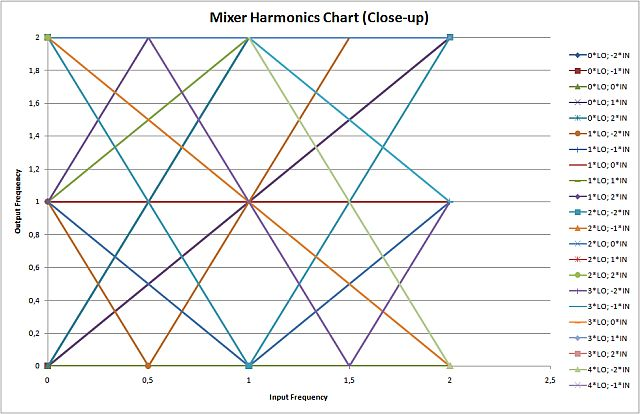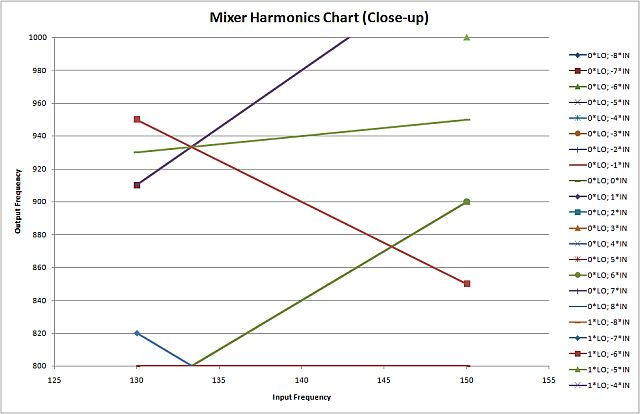# Mixer Spur Chart

This page supports an Excel spreadsheet you can retrieve from our download area, it is called Mixer.xls. Most of the material below was contributed back in 2009 by Gerald who offers consulting services with emphasis on training, This email address is being protected from spambots. You need JavaScript enabled to view it. if you want to call or email him!

### Introduction

The Mixer Spurious chart is a graphical representation of wanted and unwanted (spurious) mixing products in-band and out-of-band. Its main use is found in conjunction with mixers, however, it can be applied to all kinds of nonlinear elements, e.g. power amplifiers.

It is well-known (at least to those people that found their way to this web-site) that two signals at two frequencies applied to a nonlinear elements generate additional signals at predetermined frequencies. Let's consider (just for naming and without loss of generality) the case of an up-converter. Then, when applying signal one (intermediate frequency) at f_{if} and signal two (local oscillator) f_{lo}, at the output (or - if not filtered - rather at any port) the mixing signals at f_{out}=n*f_{lo}+m*f_{if} arise. n and m are any integer number. For an up-converter n=m=1, for a down-converter it would be -n=m=1 (both non-inverting). But there is more!

1. f_{if} is normally a band, not a single frequency (who would have use in communications to up-convert just a sine wave without information on it?)
2. What if n and m are not one? Where do all these frequencies go? Do they disturb us and others, or can we even use them?

To answer the latter question one could sit down on a cold and windy day, take a sheet of paper and do all the math.... or else one could also depict this graphically, preferably on a computer. For instance f_{out} is the y-axis and f_{if} is on the horizontal. Let's look at the example again:

• f_{out}=1*f_{lo}+1*f_{if} for fixed LO and swept IF (from zero to where-ever) is a straight line starting at f_lo and having a slope of one
• f_{out}=1*f_{lo}-1*f_{if} also starts on y-axis at f_{LO}, but now decreases with slope 1 relative with f_{if}
• f_{out}=n*f_{lo}+f_{if} gives us just a set of all parallel slope-one lines starting at different multiples of f_{lo}
• One more: f_{out}=f_{lo}+m*f_{if} again is a set of straight lines, now all starting at f_{lo} and having different slopes as defined my m
• I hope it was not too trivial so far, you can do the rest...

### The standard form of Spur Chart: normalized

For the LO and IF Frequency range of zero to two and for n=0,1,2,3,4 and m=-2,-1,0,1,2 you'll find all the lines in Figure 1.Figure 1. Simple Spur Chart

Of course this (ridiculous) frequency range makes sense, when we start talking about normalized frequencies...

Let's look at it more closely

• There is a horizontal line at output of 1. This is the LO, nothing else.
• The diagonal from (0,0) to (2,2) is the IF itself
• The greenish line from (0,1) to (1,2), that is usual non-harmonic up-conversion. And dark blue from (1,0) to (2,1) of course is non-inverting down-conversion.
• Other lines tell you where the higher order harmonics of IF go.
• And again one more: The falling diagonal (0,2) to (2,0), that is inverting up-conversion of the IF at double the LO, so some kind of subharmonic mixing....

Now, this is essentially all you need, just normalize your frequencies (maybe to LO) and check out the chart, check out the box of input and output signals that are of interest to you and you'll see who else might spit into it, that you may want to consider for signal integrity as well.

One use of this normalized chart is demonstrated in the "Watkins-Johnson Technote" "Mixers in Microwave Systems Part 1" by Bert C. Henderson.  This link keeps moving around and is likely to move some more now that TriQuint and rfmd have merged... As of September 2014 it worked. When you click the link the doc will download, it won't just appear in your browser...

### Closer to the "real world" and easier to read

Of course it's much easier, when you can just plug in your numbers and frequencies and get YOUR output for immediate use. So, take the GSM band (925-960 MHz) as output that was generated by up-conversion at a 800 MHz LO-driven mixer. The question is: what else happens to be in the band between 800 and 1000 MHz, more specifically at the RX-band between 880 and 915 MHz. Figure 2 gives the answer.Figure 2. GSM band example

You'll find that there are few fairly high order spurs with 2LO-5IF (red) and 6IF (green) between 880 MHz and 915 MHz. So nothing to worry much about.

If your find this example too esoteric, please suggest a better one!

### How to use the Mixer.xls Excel spreadsheet

This program calculates harmonic combinations of input signals at two different frequencies. This is particularly important for mixer applications to:

• Optimize subharmonic use
• Make sure all frequency-multiples and images are properly taken care of

You must have macros enabled for the program to work. It should be compatible with Excel 2003 and later revisions.

This was developed with the German Excel (so e.g. the decimal separator is "," (not ".")). You might run into trouble with using frequencies like 10,1 (10.1), if you encounter this do two things: 1. Let me know, I will fix it (somehow), 2. use integer number frequencies.

Manual and hints for usage for a more general explanation see above.

1. General
This little program creates the spur chart (also called web) for mixers, or generally any nonlinear device.
2. Up-converter (check for spurious emissions):
Enter the desired LO-frequency, put in the IF (or BB) frequency band as input. Depending on your power conditions (not calculated here), select the number of orders you want to get displayed. Get the desired information from the diagram, the parameters for output frequency serve to generate a zoom-window for your diagrams, that are hopefully less crowded.
3. Down-converter (check for possible spurs you may want to take care of):
Enter the desired LO-frequency and the RF-frequency and see the diagrams develop.
4. Check for possible additional bands that get mixed to your IF. Enter the LO-frequency and - as input - the desired IF-Band (so effectively using same input procedure as for the up-converter). See (Y-axis is not really the input frequency) what signals get mixed down to your IF (X-axis).
5. (Sub-harmonic) mixing (e.g. for spectrum analyzer applications):
where the IF is fixed and the LO sweeps: Put in your desired IF as the LO-frequency. This assumes that the IF is a fixed quantity, which it is for most heterodyne receiver applications. For homodyne receiver plug in zero. Put in the sweep-range of your LO as input frequencies. Make sure to understand the selection of orders to be displayed. Since roles of LO and RF have been changes, the IN-order is now effectively the LO-order, you might want to increase this number now in order to get the mixer to sub-harmonic. In the diagram you find on the Y-axis the convertible RF-bands and respectively on the X-axis the corresponding LO-frequencies. If you want to try this out, you find a good description for spectrum analyzers in Agilent's (now Keysight's) App-Note 150 "Spectrum Analysis Basics" pp.80.

After you plugged in your numbers and hit the start button the macro will generate two views:

• One full view on the entire frequency spectrum that give you the chance for an overall sanity check.
• One zoom into the frequency range that you specified as of interest to you. Here you can determine by the lines what else you need to take care of.

Good luck!

Author : Unknown Editor